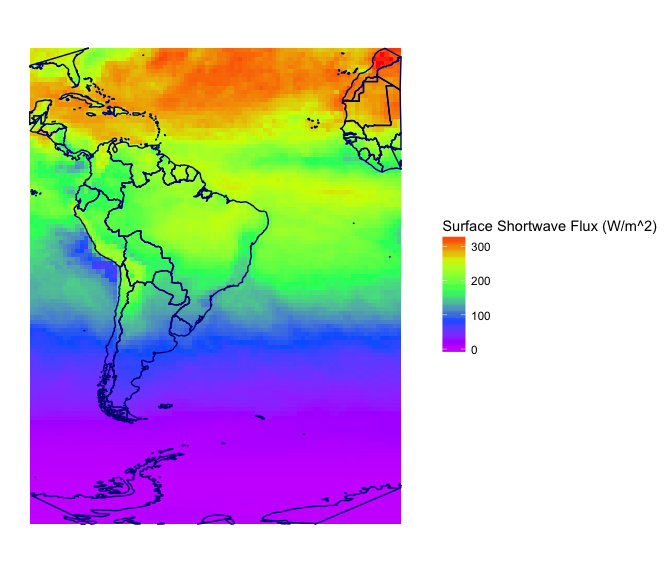# subset gridded data as geotiff and plot

#### 06 June, 2017

geoknife has a number of output formats, but a new one that was added in version 1.1.0 of the package (after the initial release to CRAN) is the ability to output results as a zip file that contains a series of geotiffs for each timestep requested. This vignette is a brief introduction on how to do this using a few additional packages that are in the Suggests field in the description.

## get the data

see the geoknife getting started vignette for more details, but for the purposes of this vignette, we need to get some data first and then plot it up.
First things first, load up the geoknife package

library(geoknife)

For this example, I am going to use a dataset that is hosted by NASA, and includes a number of radiation estimates for various components. There is a lot of global data here, but I am just going to pluck a subset in time (one timepoint for the month of July of one year) and a spatial subset. I am going to use the OUTPUT_TYPE='geotiff' argument that was added in version 1.1.0 of geoknife. We are relying on a NASA dataset here, so apologies if they change the url in the future and this doesn’t work…

knife <- webprocess(algorithm = list('OPeNDAP Subset'="gov.usgs.cida.gdp.wps.algorithm.FeatureCoverageOPeNDAPIntersectionAlgorithm"))
fabric <- webdata(url='dods://opendap.larc.nasa.gov/opendap/hyrax/SortByProduct/CERES/EBAF/Surface_Edition2.8/CERES_EBAF-Surface_Edition2.8_200003-201506.nc',
variable="sfc_sw_down_all_mon", #Surface Shortwave Flux Down, Monthly Means, All-Sky conditions
times=c('2014-07-15','2014-07-15'))
stencil <- simplegeom(data.frame('point1' = c(-5,32), 'point2' = c(-90,-78))) # big 'ol chunk 'o data
job <- geoknife(stencil, fabric, knife, wait = TRUE, OUTPUT_TYPE = "geotiff")

now that the job is complete (because wait=TRUE was used), we can download the result and unzip it:

file <- download(job, destination = file.path(tempdir(), 'nasa_data.zip'), overwrite=TRUE)
unzip(file, exdir=file.path(tempdir(),'NASA'))
tiff.dir <- file.path(tempdir(),'NASA')

## plot the data

using the rasterVis package, read this in and create a raster object:

library(rgdal)
library(rasterVis)
nasa <- raster(file.path(tiff.dir , dir(tiff.dir)))

Now, use ggplot2 and ggmap to plot this as a map:

library(maps)
library(ggmap)
library(ggplot2)

world <- map_data("world2")
gplot(nasa, maxpixels = 5e5) +
geom_tile(aes(fill = value), alpha=1) +
geom_polygon(data=world, aes(x=long, y=lat, group=group), color="navy", fill='transparent') +
scale_fill_gradientn("Surface Shortwave Flux (W/m^2)", colours=rev(rainbow(5))) +
coord_equal() +
theme_classic() +
theme(axis.line = element_blank(), axis.text.x = element_blank(), axis.text.y = element_blank(),
axis.ticks = element_blank(), axis.title.x = element_blank(), axis.title.y = element_blank()) +
scale_y_continuous(limits=c(nasa@extent@ymin, nasa@extent@ymax)) +
scale_x_continuous(limits=c(nasa@extent@xmin, nasa@extent@xmax))